## How to add percentages together:

1. Add the given percentages to 100.
2. Convert the percentages to decimals.
3. Multiply to the base value.
4. Multiply the second percentage.

We’ll go through these stages in detail below.

## Step 1: Add the given percentages to 100

For example, if we want to increase 300 by 10% then increase the result by 20%.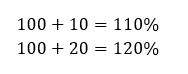## Step 2: Convert the percentages to decimals

Change the percentages to decimal by moving two decimal places to the left of the percentages.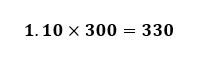## Step 3: Multiply to the base value

Multiply the first given percentage (in decimal form) to the base value. So, for our example, we multiply 300 by 1.10.## Step 4: Multiply the second percentage

Use the new value and multiply the second percentage (also in decimal form). To find the final value, we multiply 330 by 1.20.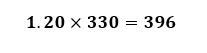## Examples of adding consecutive percentages together:

Q1) A store sells a pair of pants at a profit of 15%. A concessionaire also adds 5% on top of the cost. If the supplier cost of the pants is \$800, how much would a customer have to pay at a concessionaire?

Add the percentage with 100 first. Hence, we have: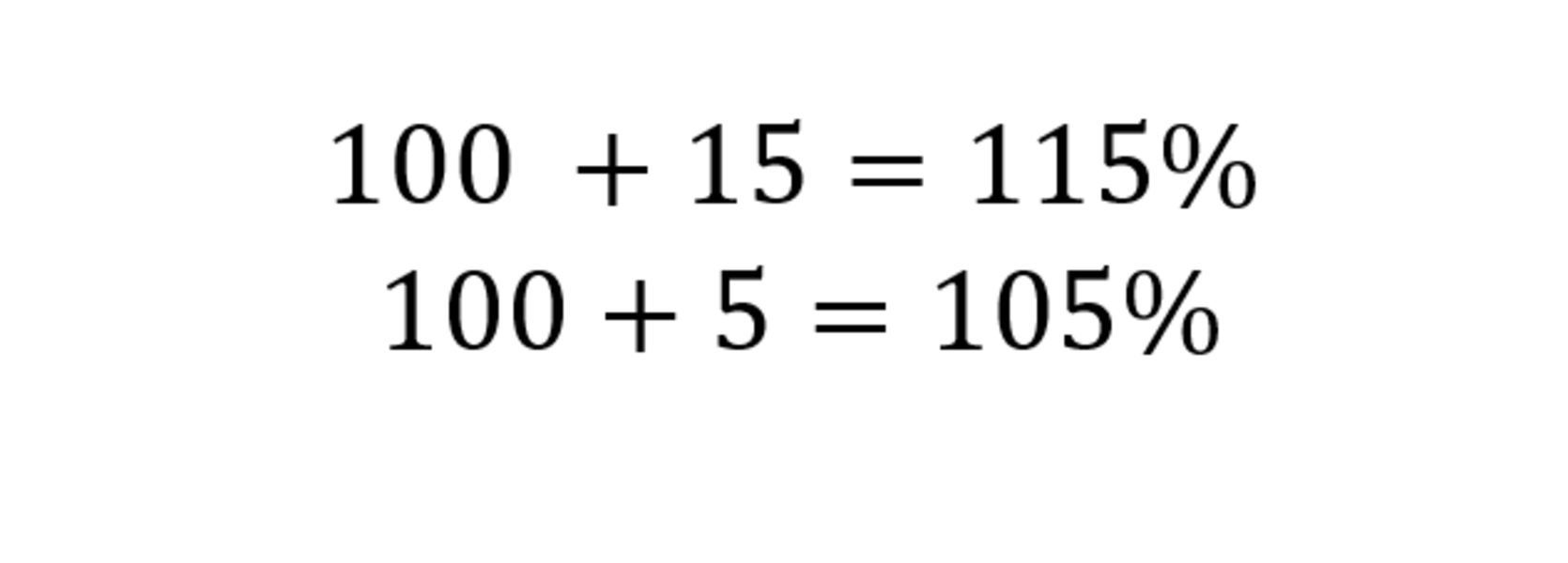Move the percentages two decimal places to the left.Now that we have the decimal forms, we first multiply 800 by 1.15.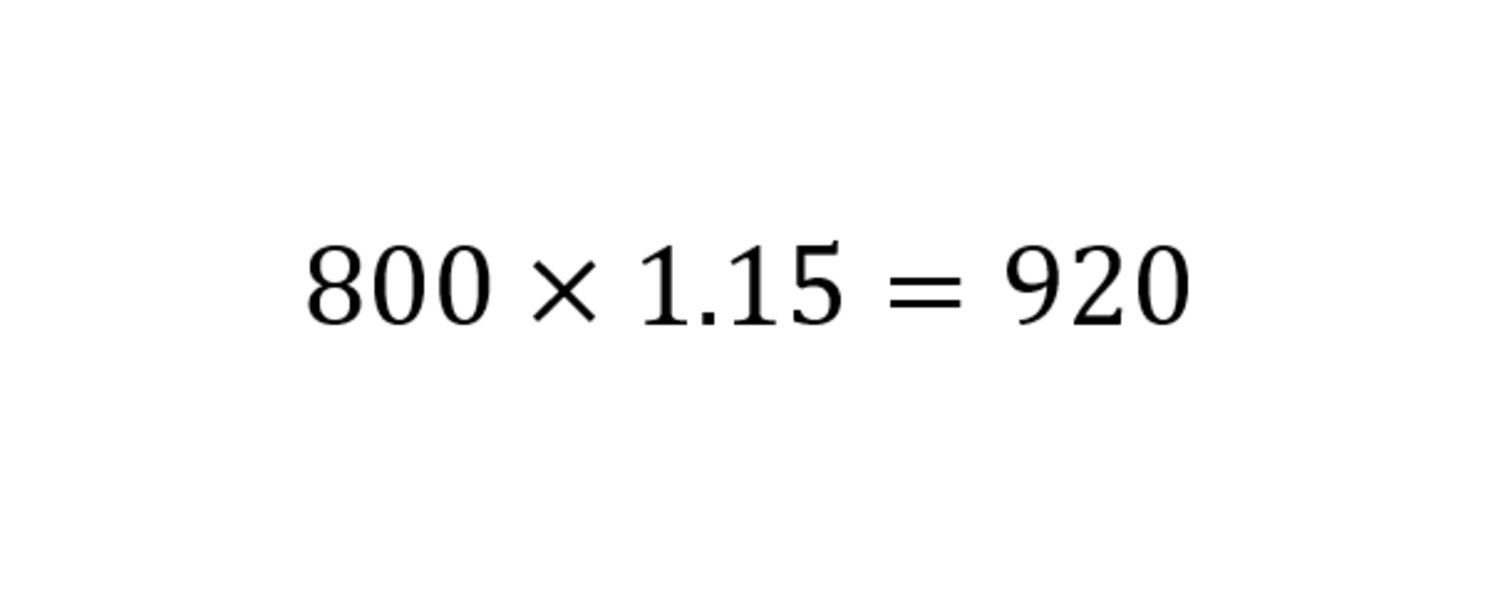To find the final cost, we multiply 920 by 1.05.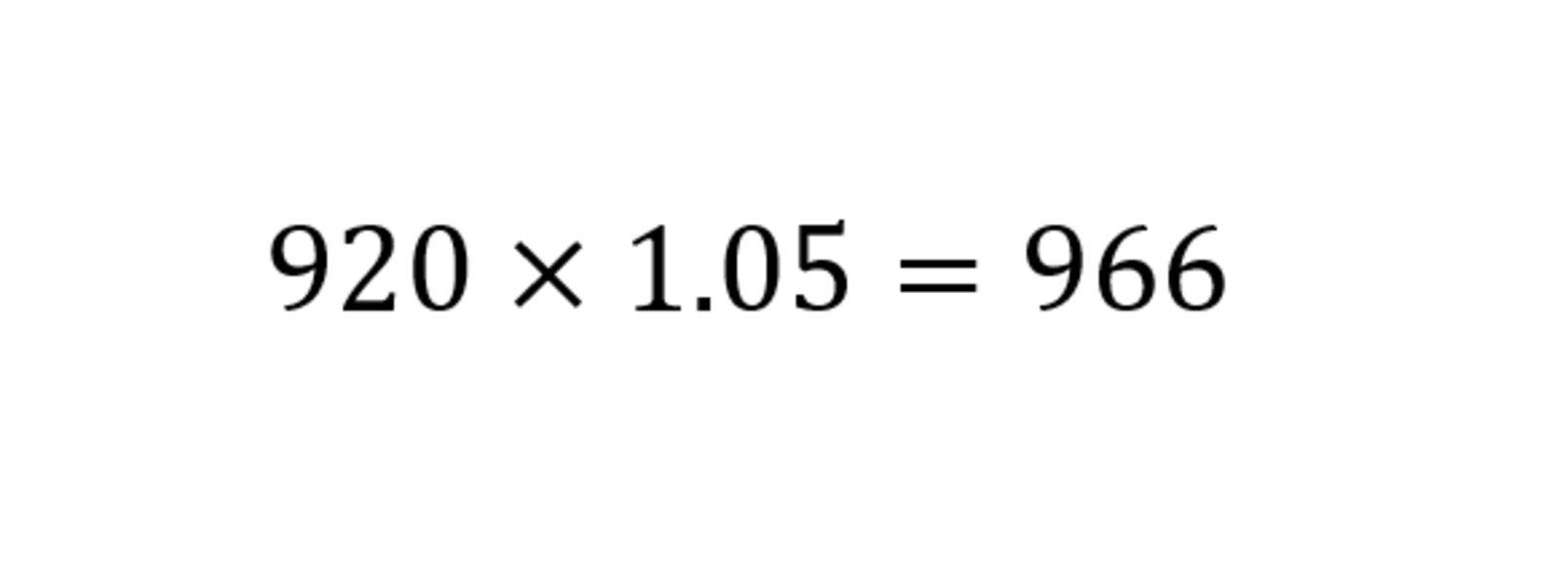So, the final cost of the pants would be \$966 at the concessionaire.